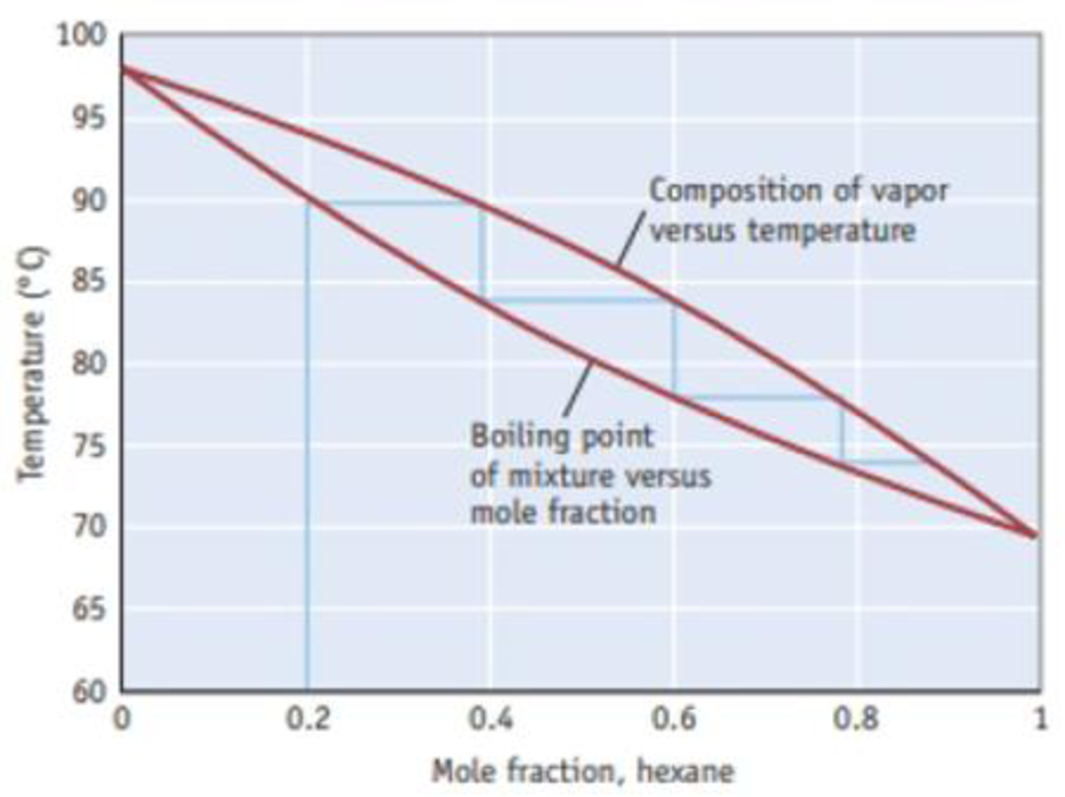Chapter 13.5, Problem 1.3ACP

Chapter
Section
Textbook Problem

What is the mass percent of hexane in a mixture with heptane if the mole fraction of hexane is 0.20?Figure B A temperature-composition diagram for hexane (C6H14)-heptane (C7H16) mixtures.

Interpretation Introduction

Interpretation: it should be determined that the mass percent of hexane in a mixture with heptane if the mole fraction of hexane is 0.20.

Concept introduction:

• Mole fraction is one of the concentrations expressing term which is equal to the number of moles of a component divided by the total number of a solution. The mole fraction of all components of a solution, when added together will equal to 1

The mole fraction of component a can be determined by using the equation,

Molefractionofa(Xa)=MoleofaTotalmoles

• Percentage by mass: ratio of amount of solute to amount of solution and multiplied with 100.

percentbymass=amountofsoluteamountofsolute+amountofsolvent×100%

• Massofthesubstance=Amountofthesubstance×Molarmass
Explanation

Mass of hexane is 17.2g and it is obtained by multiplying the number of moles of hexane with its molar mass (molar mass of hexane is 86.18g/mol ).

Mass of hexane C6H14 =0.2mol×86.18g/mol=17.2g

The mole fraction of all components of a solution, when added together will equal to 1.

Therefore the number of mole of heptane C7H16 is 1-0.20=0.8mol

Mass of heptane C7H16 =0.8mol×100

Still sussing out bartleby?

Check out a sample textbook solution.

See a sample solution

The Solution to Your Study Problems

Bartleby provides explanations to thousands of textbook problems written by our experts, many with advanced degrees!

Get Started

Behavior change is a process that takes place in stages. T F

Nutrition: Concepts and Controversies - Standalone book (MindTap Course List)

What does the size of the star image in a photograph tell you?

Horizons: Exploring the Universe (MindTap Course List)

Complete the following reactions: a. b.

Chemistry for Today: General, Organic, and Biochemistry